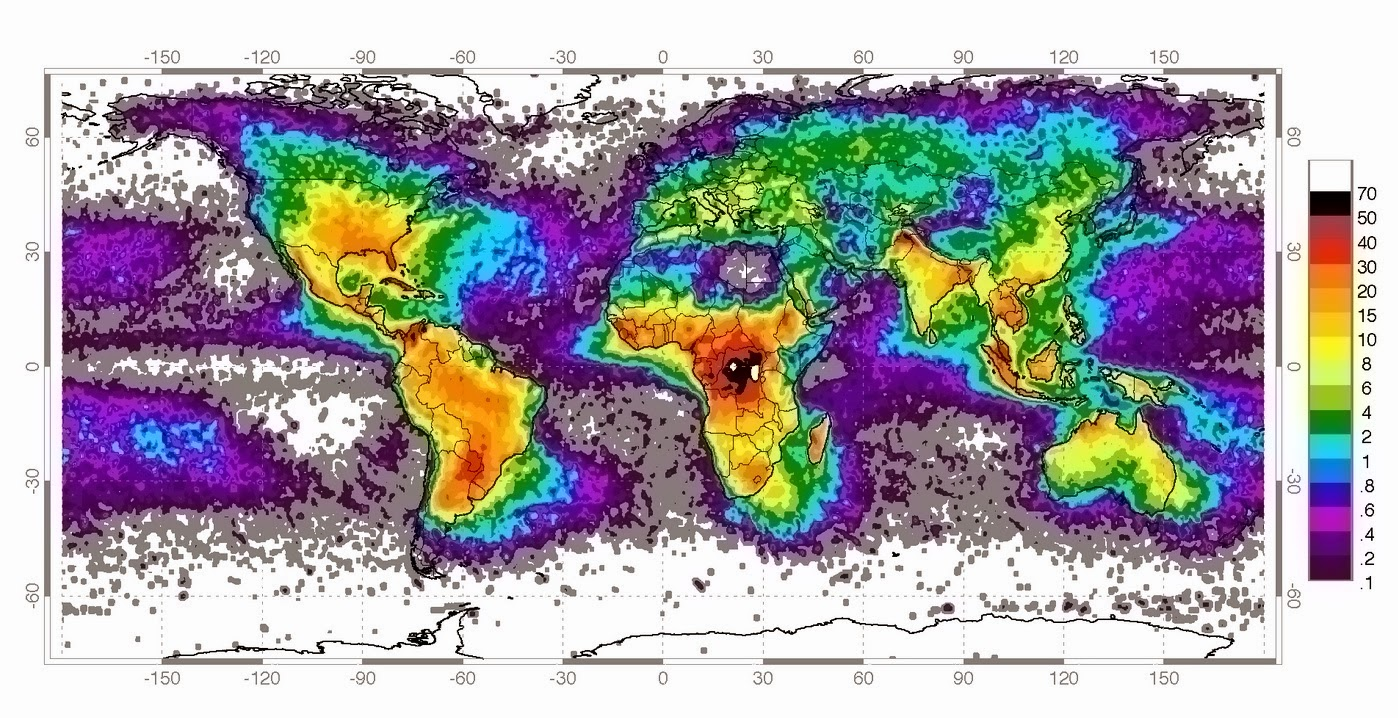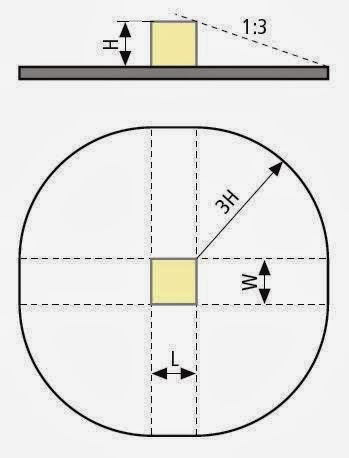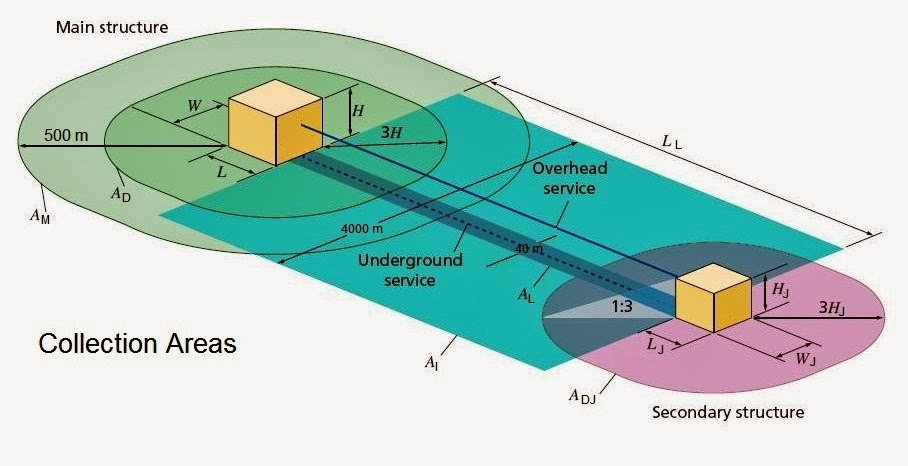# Design Calculations of Lightning Protection Systems – Part Four

In Article Design Process for Lightning Protection Systems ", I indicated the (3) phases of the Design Process for Lightning Protection Systems as follows:

 Design Process For Lightning Protection Systems The design process of lightning protection systems is commonly broken into discrete phases, allowing the lightning protection designer to present an integrated design package. These phases can be listed as follows: Planning phase, Consultation phase, Detailed Design phase. A Quality assurance is required in each phase in above.

Also, in Article Design Calculations of Lightning Protection Systems – Part One ", I explained an Introduction to design calculations of lightning protection systems as follows:

 Introduction To Design Calculations Of Lightning Protection Systems It is very important before explaining the design calculations of lightning protection systems to highlight some important topics or expressions that will be used in these calculations. These topics can be listed as follows: Sources and Types of Damage to a Structure, Types of Loss, Types of Risks Associated with Losses, Lightning Protection Levels (LPL), Lightning Protection Zones (LPZ), Class of LPS, Protection Measures.

And in Article Design Calculations of Lightning Protection Systems – Part Two ", I explained the following:

 Design Calculations of Lightning Protection Systems – Continued Third: Detailed Design Phase

The lightning protection design process involves a number of design steps as in Fig.1.Fig.1: The Lightning Protection Design Process

 Step#1: Characteristics of the Structure to Be Protected Explained in Article " Design Calculations of Lightning Protection Systems – Part Two ".

 Step#2: Risk Assessment Study

 Methods Of Calculations For Risk Assessment Study The risk assessment study can be done by (4) different methods as follows: 1- Manual Method (equations and tables method),which will be explained as per: IEC 62305-2, NFPA780. 2-Software Method, 3- Excel Sheets Method, 4-Online Calculators Method.

 First: Manual Method (Equations And Tables Method) as per IEC 62305-2

 Procedure For Performing The Risk Assessment Study By Manual Method Procedure for performing the risk assessment study includes three parts as follows: Part#1: evaluating Need for lightning protection, Part#2: Determination of Required Protection Level, Part#3: evaluating the cost-effectiveness of protection measures.

Part#1: Evaluating Need For Lightning Protection

To evaluate the need for lightning protection, the following steps need to be carried out a follows:

Step#2-1: Identify the structure to be protected.

Step#2-2: Identify the types of loss relevant to the structure to be protected Rn, where:

R1 risk of loss of human life,
R2 risk of loss of services to the public,
R3 risk of loss of cultural heritage.

Step#2-3: For each loss to be considered, identify the tolerable level of risk RT (tolerable means still acceptable).

Step#2-4: For each type of loss to be considered , identify and calculate the risk components Rx that make up risk Rn which are: RA, RB, RC, RM, RU, RV, RW, RZ.

Step#2-5: Calculate Rn = Σ Rx

Step#2-6: Comparing the calculated actual risk Rn of each loss to a tolerable level of risk (RT), then we have (2) cases:

Case#1: If the calculated risk Rn is equal or less than the respective tolerable risk RT i.e. Rn ≤ RT , then Structure is adequately protected for this type of loss and no lightning protection is required for this type of loss,
Case#2: If the calculated risk Rn is higher than the tolerable risk RT i.e.  Rn > RT, then Install lightning protection measures in order to reduce Rn.

Step#2-7: go back to step#2-4 and make a series of trial and error calculations until the risk Rn is reduced below that of RT (Rn ≤ RT).

Note:

In cases where the risk cannot be reduced to a tolerable level, the site owner should be informed and the highest level of protection provided to the installation.

The following flow diagram in Fig.2 shows this procedure for evaluating Need for lightning protection.Fig.2: Procedure For Evaluating Need For Lightning Protection

And In Article " Design Calculations of Lightning Protection Systems – Part Three ", I explained the following:

• Step#2-1: Identify the structure to be protected,
• Step#2-2: Identify the types of loss relevant to the structure to be protected Rn,
• Step#2-3: For each loss to be considered, identify the tolerable level of risk RT,
• Step#2-4 First Part: Identification of the Risk Components Rx.

Today, I will explain Step#2-4 Second Part: Calculations of the Risk Components Rx.

 Step#2-4: For each type of loss to be considered , identify and calculate the risk components Rx that make up Primary risk Rn

Second Part: Calculations of the Risk Components RxFig.3: Types Of Loss And Corresponding Primary Risks And Risk Components

Each of the risk components Rx ( see Fig.3) is obtained using further calculations, sub-calculations and reference tables based on the general equation:

RX = NX x PX x LX

Where

NX = number of dangerous events per year,
PX = probability of damage to structure,
LX = amount of consequent loss.
X = A, B, ...

1- The number NX of dangerous events:

• It is primarily based upon lightning ground flash density (NG) and by the physical characteristics of the structure to be protected, dimensions, length and type of service, its surroundings, connected lines and the soil. The physical dimensions of the structure and service are used to calculate the effective lightning capture area, and the ground flash density is used to determine the probable number of events per year.

2- The probability of damage PX:

• It is affected by characteristics of the structure to be protected, the connected lines and the protection measures provided. The probability of damage PX is obtained from given tables, with some simple calculations being required which will be explained later.

3- The consequent loss LX:

• It is affected by the use to which the structure is assigned, the attendance of persons, the type of service provided to public, the value of goods affected by the damage and the measures provided to limit the amount of loss. The amount of consequent loss LX is also obtained from given tables with calculations being required which will be explained later.

For evaluation of risk components RX related to lightning flashes to the structure and based on the general equation, the following relationships for each Risk Component are illustrated in the following Table-1 with their corresponding source and type of damage:

 Source of Damage Type of damage Lightning strike (with regard to the structure) Direct Indirect S1 Direct lightning strike into the structure S2 Lightning strike into the earth next to the structure S3 Direct lightning strike into the entering supply line S4 Lightning strike into the earth next to the entering supply line D1 Electric shock to living beings RA = ND x PA x LA RU = (NL + NDJ) x PU x LU D2 Fire, explosion, mechanical and chemical effects RB = ND x PB x LB RV = (NL + NDJ) x PV x LV D3 Interferences on electrical and electronic systems RC = ND x PC x LC RM = NM x PM x LM RW = (NL + NDJ) x PW x LW RZ = NI x PZ x LZ

Table-1: Risk Component Equations

Note: In many cases NDJ may be neglected.

So, The task of the risk assessment therefore involves the determination of the three parameters N, P and L for all relevant risk components. This involves establishing and determining of many Other individual parameters for each Risk Components and this will be done in next Articles.

 1- Calculations Of Number Of Dangerous Events Per Year NX Number of dangerous events per year NX depends on: The thunderstorm activity of the region where the structure is located, The structure’s physical characteristics. To calculate the number N use the following general equation: NX = NG x AD x Correction Factors Where: NG: The lightning ground flash density which is the number of lightning flashes per km2 per year. AD: An equivalent collection area of the structure, taking into account correction factors for the structure’s physical characteristics.

1.1 Calculation Of The Lightning Ground Flash Density NG

The value of NG is available from ground flash location networks Map in many areas of the world (see Fig.4).Fig.4: Lightning Ground Flash Density World Map

Note:

If a map of NG is not available, in temperate regions it may be estimated by:

NG = 0,1 TD

Where TD is the thunderstorm days per year (which can be obtained from isokeraunic maps in Fig.5).Fig.5: Isokeraunic World Map

Note:

The NG Map and isokeraunic maps may vary each year and you must get the most recent one as possible for better results.

1.2 Determination of the collection area AD

Case#1: For Isolated Structures On Flat Ground

The collection area AD is the area defined by the intersection between the ground surface and a straight line with 1/3 slope which passes from the upper parts of the structure (touching it there) and rotating around it. Determination of the value of AD may be performed graphically or mathematically as will be seen in sub-cases A and B.

A- Rectangular structure

For an isolated rectangular structure with length L, width W, and height H on flat ground, the collection area is then equal to:

AD = L x W + 2 x (3 x H) x (L + W) + Π x (3 x H)2

Where L, W and H are expressed in meters (see Fig.6).Fig.6: Collection Area AD Of An Isolated Structure

B- Complex shaped structure

If the structure has a complex shape such as elevated roof protrusions (see Fig.7), a graphical method should be used to evaluate AD (see Fig.8).Fig.7: Complex Shaped StructureFig.8: Different Methods To Determine The Collection Area For The Given Structure

An acceptable approximate value of the collection area is the greater between the collection area ADMIN evaluated with above Equation (in case of Rectangular structure) taking the minimum height HMIN of the structure, and the collection area attributed to the elevated roof protrusion AD. ADmay be calculated by:

Where HP is the height of protrusion.

Case#2: Structure as a Part of a Building

Where the structure S to be considered consists of only a part of a building B, the dimensions of structure S may be used in evaluation of AD provided that the following conditions are fulfilled (see Fig.9):

1. The structure S is a separated vertical part of the building B;
2. The building B does not have a risk of explosion;
3. Propagation of fire between the structure S and other parts of the building B is avoided by means of walls with resistance to fire of 120 min or by means of other equivalent protection measures;
4. Propagation of overvoltages along common lines, if any, is avoided by means of SPDs installed at the entrance point of such lines in the structure or by means of other equivalent protection measure.

Note:

Where these conditions are not fulfilled, the dimensions of the whole building B should be used.Fig.9: Structure to be considered for evaluation of collection area AD

1.3 The Correction Factors

The Correction Factors include the following:

1. The Location Factor CD,
2. Line installation factor CI,
3. Line type factor CT,
4. Line environmental factor CE.

A- The Location Factor CD (Relative Location Of The Structure)

The relative location of the structure, compensating for surrounding structures or an exposed location, will be taken into account by a location factor CD (see Table-2).

A more precise evaluation of the surrounding objects' influence can be obtained considering the relative height of the structure with respect to the surrounding objects or the ground within a distance of 3 x H from the structure and assuming CD = 1.

 Relative location CD Structure surrounded by higher objects 0,25 Structure surrounded by objects of the same height or smaller 0,5 Isolated structure: no other objects in the vicinity 1 Isolated structure on a hilltop or a knoll 2
Table-2: Structure location factor CD

B- Line Installation Factor CI (see Table-3)

 Routing CI Aerial 1 Buried 0,5 Buried cables running entirely within a meshed earth termination 0,01
Table-3: Line Installation Factor CI

C- Line Type Factor CT (see Table-4)

 Installation CT LV power, telecommunication or data line 1 HV power (with HV/LV transformer) 0,2
Table-4: Line Type Factor CT

D- Line Environmental Factor CE (see Table-5)

 Environment CE Rural 1 Suburban 0,5 Urban 0,1 Urban with tall buildings a 0,01 a Buildings higher than 20 m.
Table-5: Line Environmental Factor CE

 2- Calculation of the average annual number of dangerous events ND due to flashes to a structure and NDJ to an adjacent structure

2.1 Number of dangerous events ND for the structure

ND may be evaluated as the product:

ND = NG x AD x CD x 10–6

Where:

NG is the lightning ground flash density (1/km2 x year);
AD is the collection area of the structure (m2) (see Fig.10);
CD is the location factor of the structure (see Table-2).Fig.10: Equivalent Collection areas AD , AL , ADJ for direct lightning strikes into structures/ supply lines and AM , AI for indirect lightning strikes near the structures/supply lines

 2.2 Number of dangerous events NDJ for an adjacent structure The average annual number of dangerous events due to flashes to a structure connected at the far end of a line, NDJ (see Table-1 and Fig.10) may be evaluated as the product: NDJ = NG x ADJ x CDJ x CT ×10–6 Where: NG is the lightning ground flash density (1/km2 x year); ADJ is the collection area of the adjacent structure (m2) (see Fig.10); CDJ is the location factor of the adjacent structure (see Table-2); CT is the line type factor (see Table-4);

 2.3 Calculation of the average annual number of dangerous events NM due to flashes near a structure NM may be evaluated as the product: NM = NG x AM x 10-6 Where: NG is the lightning ground flash density (1/km2 x year); AM is the collection area of flashes striking near the structure (m2). The collection area AM extends to a line located at a distance of 500 m from the perimeter of the structure (see Fig.10): AM = 2 x 500 x (L + W) + Π x 5002

 2.4 Calculation of the average annual number of dangerous events NL due to flashes to a line A line may consist of several sections. For each section of line, the value of NL may be evaluated by: NL = NG x AL x CI x CE x CT x 10–6 Where: NL is the number of overvoltages of amplitude not lower than 1 kV (1/year) on the line section) NG is the lightning ground flash density (1/km2 x year); AL is the collection area of flashes striking the line (m2) (see Fig.10); CI is the installation factor of the line (see Table-3); CT is the line type factor (see Table-4); CE is the environmental factor (see Table-5); With the collection area for flashes to a line: AL = 40 x LL LL is the length of the line section (m). Where the length of a line section is unknown, LL = 1 000 m is to be assumed. Notes: National committees may improve this information in order to better meet national conditions of power and telecommunication lines. The ground resistivity affects the collection area AL of buried sections. In general, the larger the ground resistivity, the larger the collection area (AL proportional to √ρ). The installation factor of Table-3 is based on ρ = 400 Wm.

 2.5 Calculation of average annual number of dangerous events NI due to flashes near a line A line may consist of several sections. For each section of line, the value of NI may be evaluated by NI = NG x AI x CI x CE x CT x 10–6 Where: NI is the number of overvoltages of amplitude not lower than 1 kV (1/year) on the line section; NG is the lightning ground flash density (1/km2 x year); AI is the collection area of flashes to ground near the line (m2) (see Fig.10); CI is the installation factor (see Table-3); CT is the line type factor (see Table-4); CE is the environmental factor (see Table-5). With the collection area for flashes near a line AI = 4 000 x LL Where LL is the length of the line section (m). Where the length of a line section is unknown, LL =1 000 m is to be assumed. Notes: National committees can improve this information in order to better meet national conditions of power and telecommunication lines.

In the next Article, I will continue explaining Step#2-4 Second Part: Calculations of the Risk Components Rx. Please, keep following.